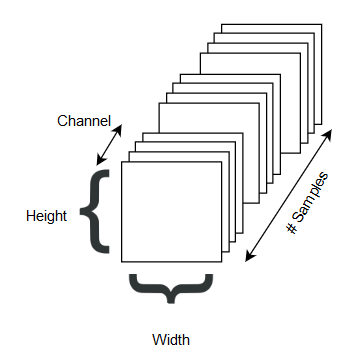# Vectors, Matrix And Tensors

Posted on Aug 01, 2020 · 5 mins read

In this post we will see how large data is stored in multi-dimensional arrays, which is also called as tensors.

Tensors are used by Machine Learning systems to store the data. Do you know Google’s Tensorflow is named after Tensors.

Tensors only stores numerical data and you have seen like for Machine learning modelling even the text data are converted to Numerical features for processing.

Tensors are like Matrices but with Arbitrary number of Dimensions.

Let’s start in this order Scalar, Vector and Matrices

## Scalar (Zero Dimensional Tensor)

Scalar is a numerical value and contains one value and thus called a Zero-Dimensional Tensor. In Numpy a float32 or float64 number is a scalar tensor.

We can use numpy’s ndim function to display the number of axes of a Numpy Tensor

``````>> import numpy as np
>> x = np.array(10)
>> x.ndmim
0
``````

## Vector (1 D Tensor)

Vector quantities are those physical quantities which are characterized by both magnitude and direction.

An Array of numbers represents a vector, which is also called as 1D Tensor.

Let’s understand this with the help of example:

``````>> import numpy as np
>> x = np.array([40, 12, 24, 8, 15, 39])
>> x.ndmim
1
``````

This array of number is a Vector with six data elements and therefore it is called a 6-dimensional vector.

For example, Consider a Person’s Id, Age, Salary, Experience, YOB as a 5D vector like [007, 42, 100000, 18, 1962] and this Vector has a single axis so a 1D Tensor. Dimensionality basically tells the number of entries along an axis.

Don’t confuse a 5D Vector with a 5D tensor because a 5D tensor will have 5 axes and represents the number of axes in a tensor

## Matrices(2D Tensor)

A Matrix has two axes rows and columns so it is a 2D Tensor because it contains two axis. The other definition is array of vectors.

``````>> import numpy as np
>> x = np.array([40, 12, 24],
[13, 29, 47],
[18, 16, 25],
[9, 8, 28])
>> x.ndmim
2
``````

So the above Matrix is a 4x3 i.e 4 rows and 3 columns. Let’s take a look at some higher dimensional matrix

``````>> import numpy as np
>> x = np.array([[[6, 25, 9, 19, 2],
[12, 25, 13, 46, 7],
[4, 9, 14, 37, 8]],
[[52, 68, 22, 9, 4],
[61, 4, 31, 68, 20],
[17, 88, 9, 7, 41]],
[[58, 12, 19, 16, 34],
[10, 4, 8, 61, 50],
[5, 15, 69, 25, 15]]])
>> x.ndmim
3
``````

This is a 3D Tensor or a Matrix of 3 Dimensions. In Deep Learning we will be manipulating such high dimension matrix through each layers

So there are two important attributes of any Tensor:

1. Axes: The axes of tensor defines what’s the dimension of a Tensor whether it is 2D or 3D Tensor.

We have seen how to get the dimension of a 1d, 2D and 3D Tensors using numpy ndim function

2. Shape: The shape of tensors define how many entries or dimensions it has along an axis.

For example a 2D Matrix shown above has a shape of 4x3 and 3D Matrix has a shape of 3x3x5.

Whereas a Scalar have a shape of Zero and the Vector has a shape of (1,5)

To make the concept of Tensors more clear we will take an example from Keras Dataset to understand that and this example is mentioned in the Deep Learning Book by Francois Chollet

Let’s load the Keras MNIST dataset:

``````from keras.datasets import mnist
(train_images, train_labels), (test_images, test_labels) = mnist.load_data()
``````

Now let’s check the number of axes in the train or test images

``````>> train_images.ndim
3
>> train_images.shape
(60000, 28, 28)
>> train_images.dtype
uint8
``````

So what does it means, This MNIST dataset is a 3D Tensor because it has 3 axes and more precisely it’s an array of 60,000 matrices of 28x8 integers. Each such matrix is a grayscale image, with coefficients between 0 and 255Images are built to have three dimensions: height, width, and color depth.

The Grayscale MNIST dataset in Keras have one single color channel. So a batch of 180 grayscale images of size 256x256 can be stored in a tensor of shape (180 , 256, 256, 1)